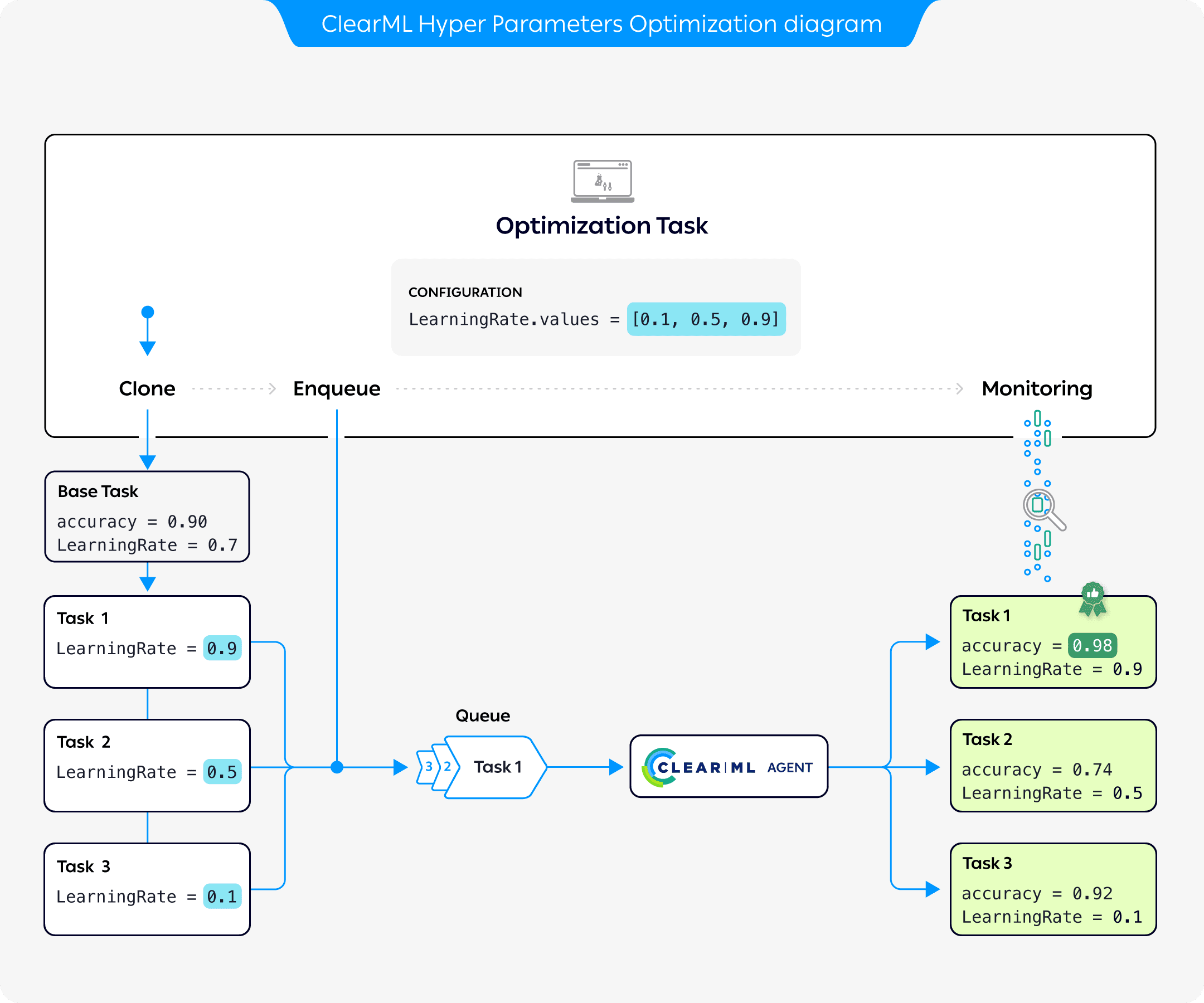# Hyperparameter Optimization

## What is Hyperparameter Optimization?​

Hyperparameters are variables that directly control the behaviors of training algorithms, and have a significant effect on the performance of the resulting machine learning models. Finding the hyperparameter values that yield the best performing models can be complicated. Manually adjusting hyperparameters over the course of many training trials can be slow and tedious. Luckily, you can automate and boost hyperparameter optimization (HPO) with ClearML's `HyperParameterOptimizer` class.

## ClearML's Hyperparameter Optimization​

ClearML provides the `HyperParameterOptimizer` class, which takes care of the entire optimization process for users with a simple interface.

ClearML's approach to hyperparameter optimization is scalable, easy to set up and to manage, and it makes it easy to compare results.

### Workflow​The diagram above demonstrates the typical flow of hyperparameter optimization where the parameters of a base task are optimized:

1. Configure an Optimization Task with a base task whose parameters will be optimized, and a set of parameter values to test
2. Clone the base task. Each clone's parameter is overridden with a value from the optimization task
3. Enqueue each clone for execution by a ClearML Agent
4. The Optimization Task records and monitors the cloned tasks' configuration and execution details, and returns a summary of the optimization results in tabular and parallel coordinate formats, and in a scalar plot.### Supported Optimizers​

The `HyperParameterOptimizer` class contains ClearML’s hyperparameter optimization modules. Its modular design enables using different optimizers, including existing software frameworks, enabling simple, accurate, and fast hyperparameter optimization.

## Defining a Hyperparameter Optimization Search Example​

1. Import ClearML's automation modules:

``from clearml.automation import UniformParameterRange, UniformIntegerParameterRangefrom clearml.automation import HyperParameterOptimizerfrom clearml.automation.optuna import OptimizerOptuna``
2. Initialize the Task, which will be stored in ClearML Server when the code runs. After the code runs at least once, it can be reproduced, and the parameters can be tuned:

``from clearml import Tasktask = Task.init(    project_name='Hyper-Parameter Optimization',    task_name='Automatic Hyper-Parameter Optimization',    task_type=Task.TaskTypes.optimizer,    reuse_last_task_id=False)``
3. Define the optimization configuration and resources budget:

``optimizer = HyperParameterOptimizer(      # specifying the task to be optimized, task must be in system already so it can be cloned      base_task_id=TEMPLATE_TASK_ID,        # setting the hyperparameters to optimize      hyper_parameters=[          UniformIntegerParameterRange('number_of_epochs', min_value=2, max_value=12, step_size=2),          UniformIntegerParameterRange('batch_size', min_value=2, max_value=16, step_size=2),          UniformParameterRange('dropout', min_value=0, max_value=0.5, step_size=0.05),          UniformParameterRange('base_lr', min_value=0.00025, max_value=0.01, step_size=0.00025),          ],      # setting the objective metric we want to maximize/minimize      objective_metric_title='accuracy',      objective_metric_series='total',      objective_metric_sign='max',        # setting optimizer        optimizer_class=OptimizerOptuna,        # configuring optimization parameters      execution_queue='default',        max_number_of_concurrent_tasks=2,        optimization_time_limit=60.,       compute_time_limit=120,       total_max_jobs=20,        min_iteration_per_job=15000,        max_iteration_per_job=150000,        )``

## Optimizer Execution Options​

The `HyperParameterOptimizer` provides options to launch the optimization tasks locally or through a ClearML queue. Start a `HyperParameterOptimizer` instance using either `HyperParameterOptimizer.start` or `HyperParameterOptimizer.start_locally`. Both methods run the optimizer controller locally. The `start` method launches the base task clones through a queue specified when instantiating the controller, while `start_locally` runs the tasks locally.

Remote Execution

You can also launch the optimizer controller through a queue by using the `Task.execute_remotely` method before starting the optimizer.

## Tutorial​

Check out the Hyperparameter Optimization tutorial for a step-by-step guide.

## Hyperparameter Optimization CLI​

ClearML also provides `clearml-param-search`, a CLI utility for managing the hyperparameter optimization process. See ClearML Param Search for more information.

## SDK Reference​

For detailed information, see the complete HyperParameterOptimizer SDK reference page.